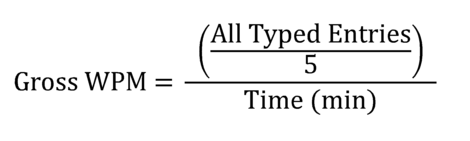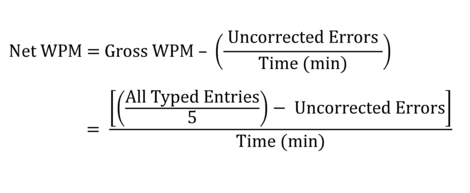OK

# How to Calculate Typing Speed (WPM) and Accuracy

The following are some of the typing equations and formulas that are used in calculating your typing statistics when you take our free typing test.

## Gross WPMGross, or Raw WPM (Words Per Minute) is a calculation of exactly how fast you type with no error penalties. The gross typing speed is calculated by taking all words typed and dividing by the time it took to type the words in minutes.

When calculating typing speed, a "word" is any five characters. For instance, "I love keyboarding, don't you?" would be counted as 6 words (30 characters / 5) and not just 5. This makes sense because typing "deinstitutionalization" obviously should count more than typing "my". Spaces, numbers, letters, and punctuation are all included, but any function keys such as Shift or Backspace are not included.

This makes the number of words easy to calculate. Simply count all typed entries and divide by five to get the number of words typed. To give an example, if you typed 200 characters in 1 minute, your net wpm typing speed would be (200 characters / 5) / 1 min = 40 WPM. If you typed 200 characters in 30 seconds your net speed would be (200/5) / 0.5 = 80 WPM.

A Net WPM calculation is preferred for measuring typing speed as opposed to the Gross WPM computation since including mistakes will give a more complete picture of your true typing abilities. However, Gross WPM is used in the calculation of the Net WPM calculation which merits its mention.

## Net WPMNet WPM is argueably the most useful tool in gauging typing abilities. Since errors play a part in its calculation, it is more a measure of typing productivity than of just typing speed. In other words, a fast but error-prone typist will receive a lower net typing speed than a slower but more accurate typist - relatively speaking of course.

This makes sense because proof-reading and correcting errors takes up more time than simply typing a passage correctly in the first place. Less mistakes also means less chance for errors being missed during proof-reading and making their way into the final product.

To calculate Net WPM, take your gross WPM result and subtract the amount of errors you left in per minute, also known as the error rate. To calculate error rate, simply divide the number of errors by the time you typed for in minutes. For example, if you typed for two minutes with a gross typing speed of 80 WPM and left in 8 mistakes, your error rate would be (8 errors / 2 mins) = 4 errors per minute. Your net typing speed would then be (80 - 4) = 76 WPM. Note that for every mistake you make per minute your typing speed goes down by 1 WPM.

This is a rather simple equation - the hard part is actually deciding which types of errors should be counted. There are two kinds of errors:

• Errors that are made and corrected
• Errors that are made and not corrected

The first impulse is to count all errors regardless of correction, however there are two strong arguments as to why only the uncorrected errors should be penalized:

1) Fixing mistakes not only messes up the typist's rhythm and likely causes brief pauses, but function keys like the delete key take time to press and do not count as a keyed entry. This means that the time penalty is already built into the process of correcting a mistake. An additional time penalty on top of the inherent time penalty discourages the typist from correcting their mistakes in the first place. While typists should be encouraged to avoid mistakes at all costs, they should also be encouraged and not discouraged from fixing mistakes as they are typing. Errors left in uncorrected are much more devastating and undesirable than errors fixed immediately while typing.

2) Take the case of a large number of corrected errors. Assume a one minute test was taken with a typing speed of 20 WPM. 20 errors were made during the test but all of them were immediately corrected. If we then deduct the error penalty considering all errors, we calculate the net speed as 0 WPM! An error-free length of text was obviously typed (20 * 5 - 20 = 80 characters to be exact), yet a typing speed of 0 WPM suggests nothing was ever typed. If the mistakes were left uncorrected, obviously one-fifth of a passage typed incorrectly would be a blunder to proofread and 0 WPM would be acceptable since the resulting text would be useless. The typist is still encouraged to reduce errors because obviously they would have typed faster than 20 WPM if they hadn't wasted time correcting 20 errors!

These two points more than prove that only errors left uncorrected should be penalized.

## Accuracy

Typing accuracy is defined as the percentage of correct entries out of the total entries typed. To calculate this mathematically, take the number of correct characters typed divided by the total number, multiplied by 100%. So if you typed 90 out of 100 characters correctly you typed with 90% accuracy.

It is interesting to note that all errors, whether corrected or not, should be counted in the accuracy calculation, unlike the net WPM calculation. This is because the calculation is more "live" than typing speed and literally describes the likelihood that the next character will be typed correctly, regardless of whether it will be corrected or not.